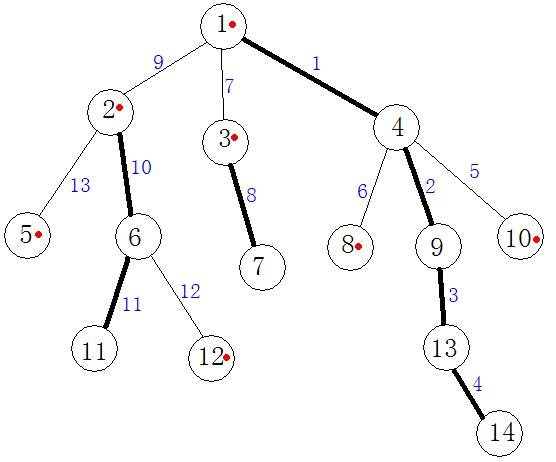树链剖分教程 & bzoj 1036 [ZJOI2008] 树的统计 Count 题解

【原题】

1036: [ZJOI2008]树的统计Count

Time Limit: 10 Sec  Memory Limit: 162 MB
Submit: 4465  Solved: 1858
[Submit][Status]

4
1 2
2 3
4 1
4 2 1 3
12
QMAX 3 4
QMAX 3 3
QMAX 3 2
QMAX 2 3
QSUM 3 4
QSUM 2 1
CHANGE 1 5
QMAX 3 4
CHANGE 3 6
QMAX 3 4
QMAX 2 4
QSUM 3 4

4
1
2
2
10
6
5
6
5
16

Source

【序言】我可是用树链剖分做的= =树分治写起来更麻烦。树剖我今天刚刚入门= =，写一篇题解加深印象。

【问题】在一棵树上进行路径的修改、求极值、求和。

【树链剖分的概念】树链，就是树上的路径。剖分，就是把路径分类为重链轻链。树链剖分就是把一些点合成一条路径，使其在线段树中的编号（下标）有序，并用线段树来维护，使得查询、修改的效率大大提高（有点像莫队的分块思想）。假设我们把路径分好链了（先不要在乎是怎么分的），每次询问两个点对（x，y）时，若x和y在同一链中，直接询问线段树中的u和v（因为同一条链中下标是连续的）u，v是x，y对应的线段树中的点。否则的话，我们从深度大的点上一点一点向上爬，每次记录该点所在的链上的情况，直到x，y在同一条链上。

【注意】树链剖分中的线段树中每个点代表的意义可以是原图的边或点。这道题是点，我就以点来叙述。

【数组含义简介】记num[v]表示以v为根的子树的节点数，deep[v]表示v的深度(根深度为1)，top[v]表示v所在的链的顶端节点，f[v]表示v的父亲，son[v]表示与v在同一重链上的v的儿子节点（姑且称为重儿子），tree[v]表示节点v在线段树中的编号，pre[v]表示线段树中编号是v的节点所对应的原图中的点（与tree相反）

【术语解释】
重儿子：num[u]为v的子节点中num值最大的，那么u就是v的重儿子。
轻儿子：v的其它子节点。
重边：点v与其重儿子的连边。
轻边：点v与其轻儿子的连边。
重链：由重边连成的路径。
轻链：轻边。
剖分后的树有如下性质：
性质1：如果(v,u)为轻边，则siz[u] * 2 < siz[v]；
性质2：从根到某一点的路径上轻链、重链的个数都不大于logn。

至于证明吧，我就不证了~~（其实我不会）只要能应用、并知道复杂度就行了。

【预处理算法实现】
我们可以用两个dfs来求出fa、deep、num、son、top、tree、pre。
dfs_1：把fa、deep、num、son求出来，比较简单。
dfs_2：①我们依次标记tree[v]（按搜索的顺序），同时得到pre。

②对于v，当son[v]存在（即v不是叶子节点）时，显然有top[son[v]] = top[v]。（没有就退出）

③然后我们先搜索v的重儿子u，并把u的重儿子、重孙子...的top值也置为top[v]；

④接着我们再搜索v的轻儿子u，并把u的重儿子、重孙子...的top值置为u；

将树中各边的权值在线段树中更新，建链和建线段树的过程就完成了。

【查询&修改算法实现】

例如将u到v的路径上每条边的权值都加上某值x。
记f1=top[u]，f2=top[v]。
当f1<>f2时：不妨设dep[f1]>=dep[f2]，那么就更新u到f1的权值(logn)，并使u=f[f1]。
当f1=f2时：u与v在同一条重链上，直接更新u到v路径上的点的权值(logn)，修改完成；
重复上述过程，直到修改完成。假设我们要修改11到10的路径时。
我们通过11和10的不断向上爬（具体操作详见上面），使他们最终在1号点相交。这样，11只经过重链2--11,10只经过自己和链1--14，效率相当的高。（为什么觉得有点像AC自动机呢）

【回归原题】这样，原题就是裸的树链剖分了。具体注释看代码。

【代码】

#include<cstdio>
#define S 
#define T 
#define SS 
using namespace std;
struct arr{int l,r,sum,max;}a T;  //线段树
int tree S,pre S,end S,son S,f S,data S,num S,top S,deep S;
int n,i,x,y,cnt,tot,Q;
char opt;
int Max(int a,int b){return (a>b)?a:b;}
void dfs1(int k,int fa,int d)  //这一段不用解释了吧
{
deep[k]=d;f[k]=fa;num[k]=1;
{
dfs1(go,k,d+1);num[k]+=num[go];
if (!son[k]||num[go]>num[son[k]]) son[k]=go;
}
}
void dfs2(int k,int Number)
{
top[k]=Number;tree[k]=++tot; //tree[i] 节点i在线段树中的编号
pre[tree[k]]=k;              //pre[i]  线段树中点为i的对应的节点编号
if (!son[k]) return;
dfs2(son[k],Number);        //先递归重儿子，把他的孩子中的“重”部分也置为Number
{
if (go!=son[k]&&go!=f[k]) dfs2(go,go);  //递归轻儿子
}
}
void build(int k,int l,int r) //这是线段树
{
a[k].l=l;a[k].r=r;
if (l==r) {a[k].sum=a[k].max=data[pre[l]];return;}
int mid=(l+r)>>1;
build(k<<1,l,mid);build((k<<1)+1,mid+1,r);
a[k].sum=a[k<<1].sum+a[(k<<1)+1].sum;
a[k].max=Max(a[k<<1].max,a[(k<<1)+1].max);
}
void update(int k,int x,int jia)//这是线段树
{
if (a[k].l==a[k].r)
{
a[k].sum+=jia*(a[k].r-a[k].l+1);
a[k].max+=jia;return;
}
int mid=(a[k].l+a[k].r)>>1;
if (x<=mid) update(k<<1,x,jia);else update((k<<1)+1,x,jia);
a[k].sum=a[k<<1].sum+a[(k<<1)+1].sum;
a[k].max=Max(a[k<<1].max,a[(k<<1)+1].max);
}
{
if (a[k].l>=x&&a[k].r<=y) return a[k].sum;
int mid=(a[k].l+a[k].r)>>1,o=0;
a[k].sum=a[k<<1].sum+a[(k<<1)+1].sum;
a[k].max=Max(a[k<<1].max,a[(k<<1)+1].max);
return o;
}
{
if (a[k].l>=x&&a[k].r<=y) return a[k].max;
int mid=(a[k].l+a[k].r)>>1,o=-1000000000;
a[k].sum=a[k<<1].sum+a[(k<<1)+1].sum;
a[k].max=Max(a[k<<1].max,a[(k<<1)+1].max);
return o;
}
int find_max(int x,int y)
{
int f1=top[x],f2=top[y],t,ans=-1000000000;//注意ans要置为-INF
while (f1!=f2)
{
if (deep[f1]<deep[f2]) t=f1,f1=f2,f2=t,t=x,x=y,y=t;
x=f[f1];f1=top[x];
}
return ans;
}
int find_sum(int x,int y)
{
int f1=top[x],f2=top[y],t,ans=0;
while (f1!=f2)
{
if (deep[f1]<deep[f2]) t=f1,f1=f2,f2=t,t=x,x=y,y=t;
x=f[f1];f1=top[x];
}
return ans;
}
int main()
{
freopen("count.in","r",stdin);
freopen("count.out","w",stdout);
scanf("%d",&n);
for (i=1;i<n;i++)
for (i=1;i<=n;i++)
scanf("%d",&data[i]);
dfs1(1,0,1);dfs2(1,1);
build(1,1,n);
scanf("%d",&Q);
while (Q)
{
Q--;
scanf("%s%d%d",opt,&x,&y);
if (opt=='C') update(1,tree[x],y-data[x]),data[x]=y;
else if (opt=='M') printf("%d\n",find_max(x,y));
else printf("%d\n",find_sum(x,y));
}
return 0;
}